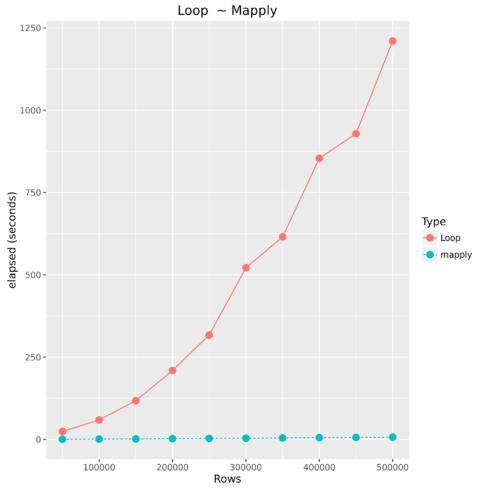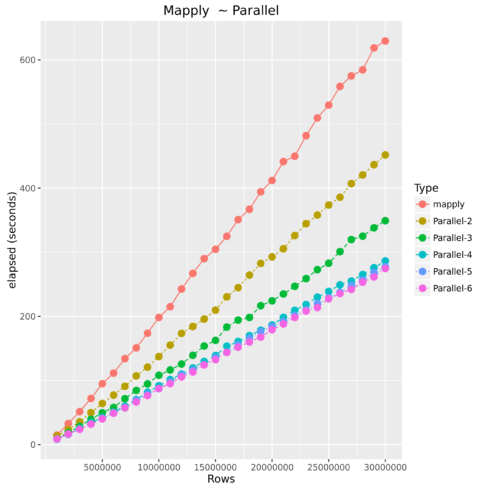Want to share your content on R-bloggers? click here if you have a blog, or here if you don't.

After using R for a few years people come to realize how inefficient loops are (in all languages), even after pre-allocating memory. Then you begin to use the apply functions and revel at their speed. However, once you have datasets > 10-15 million the apply family can begin to creep along (relative to the amount of logic in your function). Luckily, most datasets I work with are between 1 – 5 million records and do not encounter performance issues too often. However, when we do run into large datasets we have our parallel packages.

## Demo Machine

While writing this demo from my home laptop I was not seeing performance gains in parallel, because I was running out of RAM to maximize the efficiency of parallel processing. Therefore, I decided to checkout Google’s Cloud and was quite happy with the aesthetics and performance of their platform. Now, I do not work for Google, but it is worth checking out their trial.

custom (8 vCPUs, 32 GB memory)

## For Loop

Most programmers know to try and avoid loops whenever possible because of they are extremely resource intensive. However, with languages such as R, the majority of the time spent is manipulating the dataset. Oftentimes this includes cleaning and feature engineering which requires user-defined functions. If you have less than 10,000 observations and the function is not overly complex then for loops are fine. The example below processes 150 observations in three hundredth of a second.

```IrisClassifier  <- function(df,i){
if(df[i,] >= 5.8 & df[i,] >= 1.8){
return('virginica')
}else if(df[i,] <= 5.7 & df[i,] <= 1){
return('setosa')
}else{
return('versicolor')
}
}
iris\$Check <- ''
system.time(
for(i in 1:nrow(iris)){
iris\$Check[i] <- IrisClassifier(iris,i)
}
)
```
User TimeSystem TimeElapsed Time
0.030.000.03

### Vectorized Operations

How does the apply family speed up our functions? It converts a scalar (single item) process to a vector (array) process. Think of a mill having to cut lumber piece-by-piece or having one large saw and applying it to all of the lumber at once.

```IrisClassifier  <- function(col1,col2){
if(col1 >= 5.8 & col2 >= 1.8){
return('virginica')
}else if(col1 <= 5.7 & col2 <= 1){
return('setosa')
}else{
return('versicolor')
}
}

system.time(iris\$Check <- mapply(psuedoClassifier,iris[,1],iris[,4]))
```
User TimeSystem TimeElapsed Time
0.000.000.00

## Loop vs. mapply

Required Libraries:

```library(doSNOW)
library(foreach)
library(data.table)
library(sqldf)
library(ggplot2)
```

### The Dataset

For this example we will be using a simple dataset starting with 1,000,000 million observations and increment by 1,000,000 to 30,000,000 million observations. The entire script is located at the end of this example.

```df <-   df <- data.frame(a = round(rnorm(values[i],mean = 18,sd = 10),0),
b = round(rnorm(values[i],mean = 10,sd = 11),0),
c = round(rnorm(values[i],mean = 400,sd = 44),0),
d = round(rnorm(values[i],mean = 100,sd = 33),0),
e = round(rnorm(values[i],mean = 11,sd = 3),0),
f = round(rnorm(values[i],mean = 223,sd = 1000),0)
)
```

### Test Function

For this example we will use the `mapply` to show how to apply a function with multiple parameters. I find most articles online only demonstrating how to apply with one parameter so I used this as an excuse to demonstrate applying a function with multiple parameters.

```unspirited.func <- function(a,b,c,d,e,f){
if(a > 20 & b  300 & c  30 & log(c)> 6) {
return('Fail - A')
}else if(b > 38 & log(c)> 6) {
return('Fail- B')
}else if(d > 175 & log(c)> 6) {
return('Fail - D')
}else if(e > 18 & log(c)> 6) {
return('Fail - E')
}else if(f > 1989 & log(c)> 6) {
return('Fail - F')
}else{
return('Unsure')
}
}
```

### Comparing For Loop to Vectorized OperationsTypeNumber of ObservationsElapsed Time
Loop5000024.593
mapply500000.536
Loop10000059.314
mapply1000001.228
Loop150000117.658
mapply1500001.865
Loop200000209.694
mapply2000002.658
Loop250000317.212
mapply2500003.283
Loop300000521.578
mapply3000003.816
Loop350000615.212
mapply3500004.625
Loop400000854.06
mapply4000005.571
Loop450000928.809
mapply4500006.437
Loop5000001210.446
mapply5000007.179

## Mapply vs. Parallel Mapply

Parallel Operations
Luckily most current laptops, pcs, and cloud machines all offer multiple cores of processing power. Therefore, how does parallel processing work? Essentially, R starts up n number of instances and sends subsets of the original data to be processed in those instances using its own processing core, and then finally returning the results back together.TypeNumber of ObservationsElapsed Time
mapply100000014.851
Parallel-2100000013.641
Parallel-310000009.407
Parallel-410000008.741
Parallel-510000008.326
Parallel-610000008.977
mapply500000095.012
Parallel-2500000064.179
Parallel-3500000049.724
Parallel-4500000042.357
Parallel-5500000040.959
Parallel-6500000040.204
mapply10000000198.36
Parallel-210000000137.413
Parallel-310000000108.221
Parallel-41000000091.929
Parallel-51000000087.314
Parallel-61000000087.57
mapply15000000304.673
Parallel-215000000209.789
Parallel-315000000162.592
Parallel-415000000138.99
Parallel-515000000135.785
Parallel-615000000132.459
mapply20000000411.984
Parallel-220000000292.82
Parallel-320000000224.262
Parallel-420000000186.6
Parallel-520000000184.216
Parallel-620000000179.269
mapply25000000529.586
Parallel-225000000373.688
Parallel-325000000282.885
Parallel-425000000238.923
Parallel-525000000229.101
Parallel-625000000227.555
Parallel-230000000451.788
Parallel-330000000349.257
Parallel-430000000286.526
Parallel-530000000277.594
Parallel-630000000274.735

Considerations

• Required Setup time
• Parallel will end up using more RAM
• Shared server resources

## Final Note

After hearing Max Kuhn speak a few years ago he said always check to see if someone has already written the function you are coding. After, writing this post I did stumble upon some builtin in parallel apply functions. However, I have not researched exactly how these operate.

The Entire Script

```###############################################################################################################################
# Name             :  Parallel Process Example
# Date             :  2016-09-03
# Author           :  Christopher M
# Purpose          :  ~
###############################################################################################################################
# ver    user        date(YYYYMMDD)        change
# 1.0     ~          20160903              initial
###############################################################################################################################

library(parallel)
library(ggplot2)

## Display number of CPU cores on host
detectCores(all.tests = FALSE, logical = TRUE)

## Example function for processing
unspirited.func <- function(a,b,c,d,e,f){

if(a > 20 & b  300 & c  30 & log(c)> 6) {
return('Fail - A')
}else if(b > 38 & log(c)> 6) {
return('Fail- B')
}else if(d > 175 & log(c)> 6) {
return('Fail - D')
}else if(e > 18 & log(c)> 6) {
return('Fail - E')
}else if(f > 1989 & log(c)> 6) {
return('Fail - F')
}else{
return('Unsure')
}

}

## kfold function to split data for parallel processing
kfold <- function(df,k){
require(sqldf)
if( length(which(colnames(df)=='folds'))>0){
stop(print('Rename column(s) named folds'))
break

}
#randomize the data
df<-sqldf("SELECT * FROM df order by random()")

#Create equally size folds
df\$folds <- cut(seq(1,nrow(df)),breaks=k,labels=FALSE)

return(df)

}

## function for parallel processing
parallelApply <- function(df,cols,func,cores){

require(doSNOW)
require(foreach)
require(data.table)
cl <- makeCluster(cores, type="SOCK")
registerDoSNOW(cl)

## Create ID to compute in parallel
df <- kfold(df,cores)

## Process data in parallel
system.time(NewDataFrame <- foreach(i = 1:cores) %dopar% {
tmpdata <- subset(df, folds == i)
mapply(func,tmpdata[,cols],tmpdata[,cols],tmpdata[,cols],tmpdata[,cols],tmpdata[,cols],tmpdata[,cols])

})

## Bind data back into dataframe
NewDataFrame <- rbindlist(Map(as.data.frame, NewDataFrame))
colnames(NewDataFrame) <- c('ParRow')
df <- cbind(df,NewDataFrame)
stopCluster(cl)
return(df)

}

## values for dataframe length
values <- seq(1e6,30e6,1e6)

capture <- data.frame()

for(i in 1:length(values)){
print(paste("processing:",values[i],"rows. There are",length(values)-i,"iterations left to complete"))

df <- data.frame(a = round(rnorm(values[i],mean = 18,sd = 10),0),
b = round(rnorm(values[i],mean = 10,sd = 11),0),
c = round(rnorm(values[i],mean = 400,sd = 44),0),
d = round(rnorm(values[i],mean = 100,sd = 33),0),
e = round(rnorm(values[i],mean = 11,sd = 3),0),
f = round(rnorm(values[i],mean = 223,sd = 1000),0)
)
##______________________________________________________________________________
## Apply

performance <- system.time(df\$Check <- mapply(unspirited.func,df[,1],df[,2],df[,3],df[,4],df[,5],df[,6]))
capture <- rbind(capture,data.frame(Type = 'mapply',Rows = values[i],elapsed = performance,PercentDifference = 1))
df\$Check <- NULL
print('finished')
print(capture)
##______________________________________________________________________________
## Parallel Apply
base <- as.numeric(subset(capture,Rows == values[i] & Type == 'mapply'))
for(x in 2:6){
print(paste('running on',x,'cores'))
performance <- system.time(df <- parallelApply(df,c(1,2,3,4,5,6),unspirited.func,x))
capture <- rbind(capture,data.frame(Type = paste('Parallel-',x,sep=''),Rows = values[i],elapsed = performance,
PercentDifference = abs(1-(performance/base))))
df\$ParRow <- NULL
df\$folds <- NULL
}
gc()
print(capture)
}
options(scipen=999)

## Example plots
ggplot(subset(capture,Type != 'Loop'),aes(Rows,elapsed,color = Type))+
geom_line( aes(linetype=Type))+
geom_point(size=3 )+
ylab("elapsed (seconds)") +
geom_text(data=subset(capture,Rows ==max(capture\$Rows)),
aes(label=Type))+
ggtitle("Mapply  ~ Parallel")

tmp <- subset(capture,Type != 'mapply')
tmp\$PercentDifference <- tmp\$PercentDifference + 1
mapply <- subset(capture,Type == 'mapply')
capture_ <- rbind(tmp,mapply)

ggplot(subset(capture_,Type != 'Loop'),aes(Rows,PercentDifference,color = Type))+
geom_line( aes(linetype=Type))+
geom_point(size=3 )+
ylab("Performance Increase") +
ggtitle("Mapply  ~ Parallel") +
scale_x_continuous(breaks = seq(5e6,30e6,5e6))+
scale_y_continuous(breaks = seq(0,3,.1))
```

Related Post